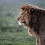## Thursday, September 12, 2013

### Collapse n random edges in a tree

"Does anyone know of an existing function that will take a resolved tree and a number (n) as input, and randomly choose n branches to collapse to zero length?"

Here is my response, for the record:

If you don't care about the keeping the total height of the tips above the root constant, you could just do:

ff<-function(tree,n){
xx<-sample(2:tree\$Nnode,n)+length(tree\$tip.label)
yy<-sapply(xx,function(x,y) which(x==y),y=tree\$edge[,2])
tree\$edge.length[yy]<-0
tree<-di2multi(tree)
tree
}
You can take out the di2multi if you want your tree to be bifurcating with internal edges of zero length.

Let's try it out:

> tree<-rtree(n=40)
> tt<-ff(tree,10)
> tt
Phylogenetic tree with 40 tips and 29 internal nodes.
Tip labels:
t12, t38, t18, t9, t7, t35, ...
Unrooted; includes branch lengths.
> par(mfcol=c(2,1))
> plotTree(tree,fsize=0.8)
> plotTree(tt,fsize=0.8)

If you want to keep the total height of the tips constant, that's going to be a little more complicated. Maybe do this, which gives all the lost branch lengths to the daughter edges (i.e., collapses down):

gg<-function(tree,n){
for(i in 1:n){
ii<-sample(2:tree\$Nnode,1)+length(tree\$tip.label)
ll<-tree\$edge.length[which(tree\$edge[,2]==ii)]
tree\$edge.length[which(tree\$edge[,1]==ii)]<-
tree\$edge.length[which(tree\$edge[,1]==ii)]+ll
tree\$edge.length[which(tree\$edge[,2]==ii)]<-0
tree<-di2multi(tree)
}
tree
}

Let's try this one:

> tree<-pbtree(n=40)
> tt<-gg(tree,10)
> tt
Phylogenetic tree with 40 tips and 29 internal nodes.
Tip labels:
t22, t25, t35, t36, t5, t4, ...
Unrooted; includes branch lengths.
> par(mfcol=c(2,1))
> plotTree(tree,fsize=0.7)
> plotTree(tt,fsize=0.7)

That's it.

1.2.